# Contents

In this vignette we demonstrate clustering of 3rd complementary determining region sequence (CDR3) and V-J gene identity of mouse T cells, ways to visualize and explore clusters that are expanded, pairing of alpha-beta clusters, tests of differential CDR3 usage, and permutation tests for overall clonal properties.

``````library(CellaRepertorium)
library(dplyr)
#>
#> Attaching package: 'dplyr'
#> The following objects are masked from 'package:stats':
#>
#>     filter, lag
#> The following objects are masked from 'package:base':
#>
#>     intersect, setdiff, setequal, union
library(ggplot2)
library(tidyr)
library(stringr)
library(purrr)``````

# 1 Load filtered contig files

We begin with a `data.frame` of concatenated contig files (‘all_contig_annotations.csv’), output from the Cellranger VDJ pipeline.

``````data(contigs_qc)
MIN_CDR3_AA = 6

cdb = ContigCellDB_10XVDJ(contigs_qc, contig_pk = c('barcode', 'pop', 'sample', 'contig_id'), cell_pk = c('barcode', 'pop', 'sample'))
cdb
#> ContigCellDB of 1508 contigs; 832 cells; and 0 clusters.
#> Contigs keyed by barcode, pop, sample, contig_id; cells keyed by barcode, pop, sample.``````

Initially we start with 832 cells and 1508 contigs. We keep contigs that are

• full - length
• productive
• high-confidence
• only from T cells
• and with CDR3 sufficiently long.

``cdb\$contig_tbl = dplyr::filter(cdb\$contig_tbl, full_length, productive == 'True', high_confidence, chain != 'Multi', str_length(cdr3) > MIN_CDR3_AA) %>% mutate( fancy_name = fancy_name_contigs(., str_c(pop, '_', sample)))``

After filtering, there are 832 cells and 1496 contigs.

# 2 Clustering contigs by sequence characteristics

As a first step to define clonotypes, we will first find equivalence classes of CDR3 sequences with the program CD-HIT. In this case, we use the translated amino acid residues, but often one might prefer to use the DNA sequences, by setting the `sequence_key` accordingly and `type = 'DNA'`. Additionally, a higher identity threshold might be appropriate (see below).

``````aa80 = cdhit_ccdb(cdb, sequence_key = 'cdr3', type = 'AA', cluster_pk = 'aa80',
identity = .8, min_length = 5, G = 1)
aa80 = fine_clustering(aa80, sequence_key = 'cdr3', type = 'AA', keep_clustering_details = TRUE)
#> Calculating intradistances on 988 clusters.
#> Summarizing``````

This partitions sequences into sets with >80% mutual similarity in the amino acid sequence, adds some additional information about the clustering, and returns it as a `ContigCellDB` object named `aa80`. The primary key for the clusters is aa80. The `min_length` can be set somewhat smaller, but there is a lower limit for the cdhit algorithm. `G=1`, the default, specifies a global alignment. This is almost always what is desired, but local alignment is available if `G=0`.

``````head(aa80\$cluster_tbl)
#> # A tibble: 6 x 4
#>    aa80 avg_distance fc               n_cluster
#>   <dbl>        <dbl> <list>               <int>
#> 1     1            0 <named list >         1
#> 2     2            0 <named list >         1
#> 3     3            0 <named list >         2
#> 4     4            0 <named list >         1
#> 5     5            0 <named list >         1
#> 6     6            0 <named list >         1
head(aa80\$contig_tbl) %>% select(contig_id, aa80, is_medoid, `d(medoid)`)
#> # A tibble: 6 x 4
#>   contig_id                    aa80 is_medoid `d(medoid)`
#>   <chr>                       <dbl> <lgl>           <dbl>
#> 1 ATCTACTCAGTATGCT-1_contig_3     1 TRUE                0
#> 2 ACTGTCCTCAATCACG-1_contig_3     2 TRUE                0
#> 3 CACCTTGTCCAATGGT-1_contig_2     3 TRUE                0
#> 4 CACCTTGTCCAATGGT-1_contig_2     3 FALSE               0
#> 5 CGGACGTGTTCATGGT-1_contig_1     4 TRUE                0
#> 6 CTGCTGTTCCCTAATT-1_contig_4     5 TRUE                0``````

The `cluster_tbl` lists the 988 80% identity groups found, including the number of contigs in the cluster, and the average distance between elements in the group. In the `contig_tbl`, there are two columns specifying if the contig `is_medoid`, that is, is the most representative element of the set and the distance to the medoid element `d(medoid)`.

``````cluster_plot(aa80)
#> `stat_bin()` using `bins = 30`. Pick better value with `binwidth`.
#> `stat_bin()` using `bins = 30`. Pick better value with `binwidth`.``````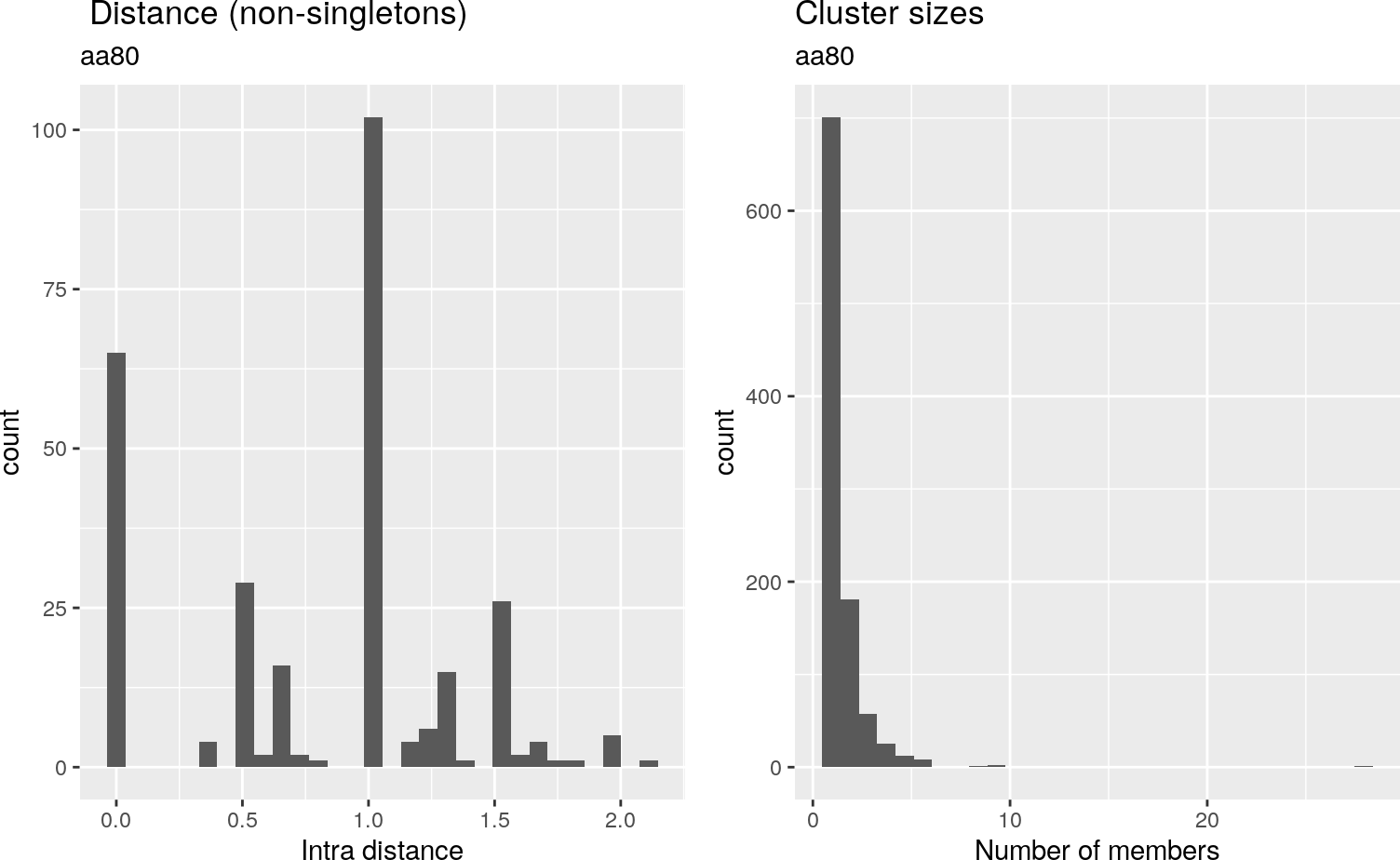## 2.1 Cluster CDR3 DNA sequences

``````cdb = cdhit_ccdb(cdb, 'cdr3_nt', type = 'DNA', cluster_pk = 'DNA97', identity = .965, min_length = MIN_CDR3_AA*3-1, G = 1)
cdb = fine_clustering(cdb, sequence_key = 'cdr3_nt', type = 'DNA')
#> Calculating intradistances on 1342 clusters.
#> Summarizing

cluster_plot(cdb)
#> `stat_bin()` using `bins = 30`. Pick better value with `binwidth`.
#> `stat_bin()` using `bins = 30`. Pick better value with `binwidth`.``````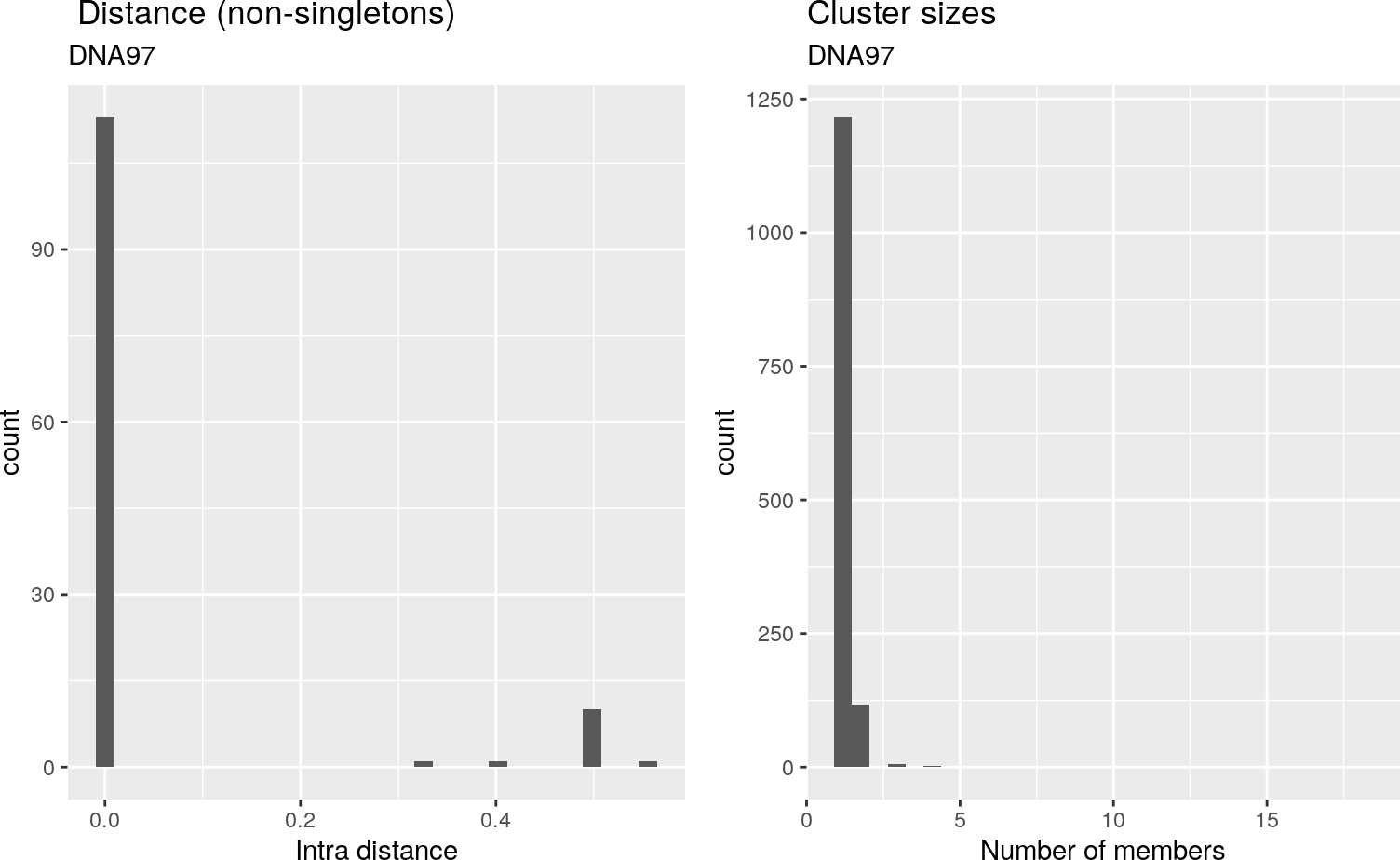We can also cluster by DNA identity.

## 2.2 Cluster by V-J identity

``````germline_cluster = cluster_germline(cdb, segment_keys = c('v_gene', 'j_gene', 'chain'), cluster_pk = 'segment_idx')
#> Warning in replace_cluster_tbl(ccdb, cluster_tbl, cl_con_tbl, cluster_pk =
#> cluster_pk): Replacing `cluster_tbl` with DNA97.``````

We can cluster by any other feature of the contigs. Here we cluster each contig based on the chain and V-J genes. This gives us the set of observed V-J pairings:

``````germline_cluster = fine_clustering(germline_cluster, sequence_key = 'cdr3_nt', type = 'DNA')
#> Calculating intradistances on 700 clusters.
#> Summarizing
#> Warning in left_join_warn(d_medoid, contig_tbl, by = ccdb\$contig_pk, overwrite =
#> TRUE): Overwriting fields d(medoid), is_medoid in table contig_tbl
ggplot(germline_cluster\$cluster_tbl %>% filter(chain == 'TRB'), aes(x = v_gene, y = j_gene, fill = n_cluster)) + geom_tile() + theme(axis.text.x = element_text(angle = 90))``````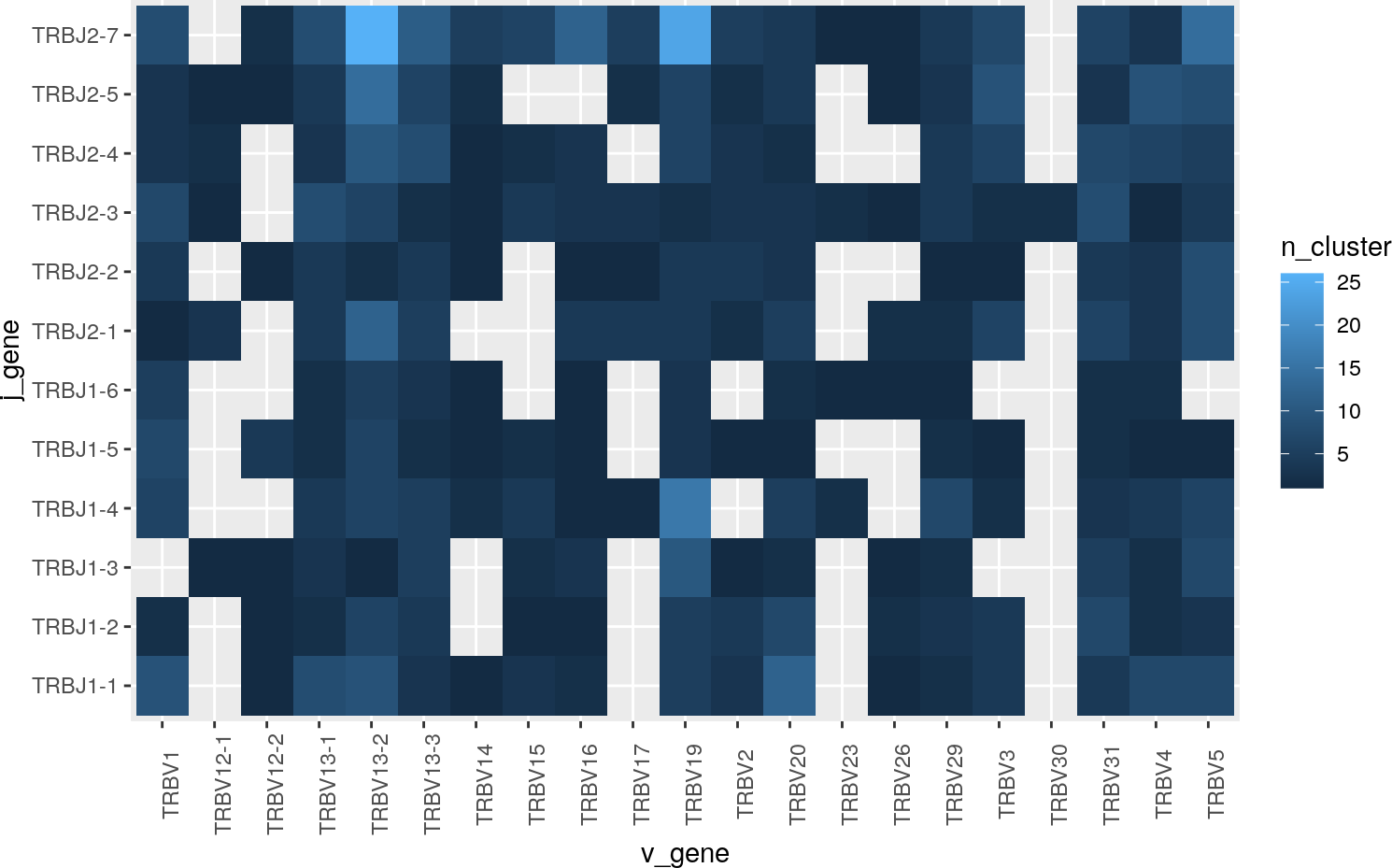Number of pairs

``ggplot(germline_cluster\$cluster_tbl %>% filter(chain == 'TRB'), aes(x = v_gene, y = j_gene, fill = avg_distance)) + geom_tile() + theme(axis.text.x = element_text(angle = 90))``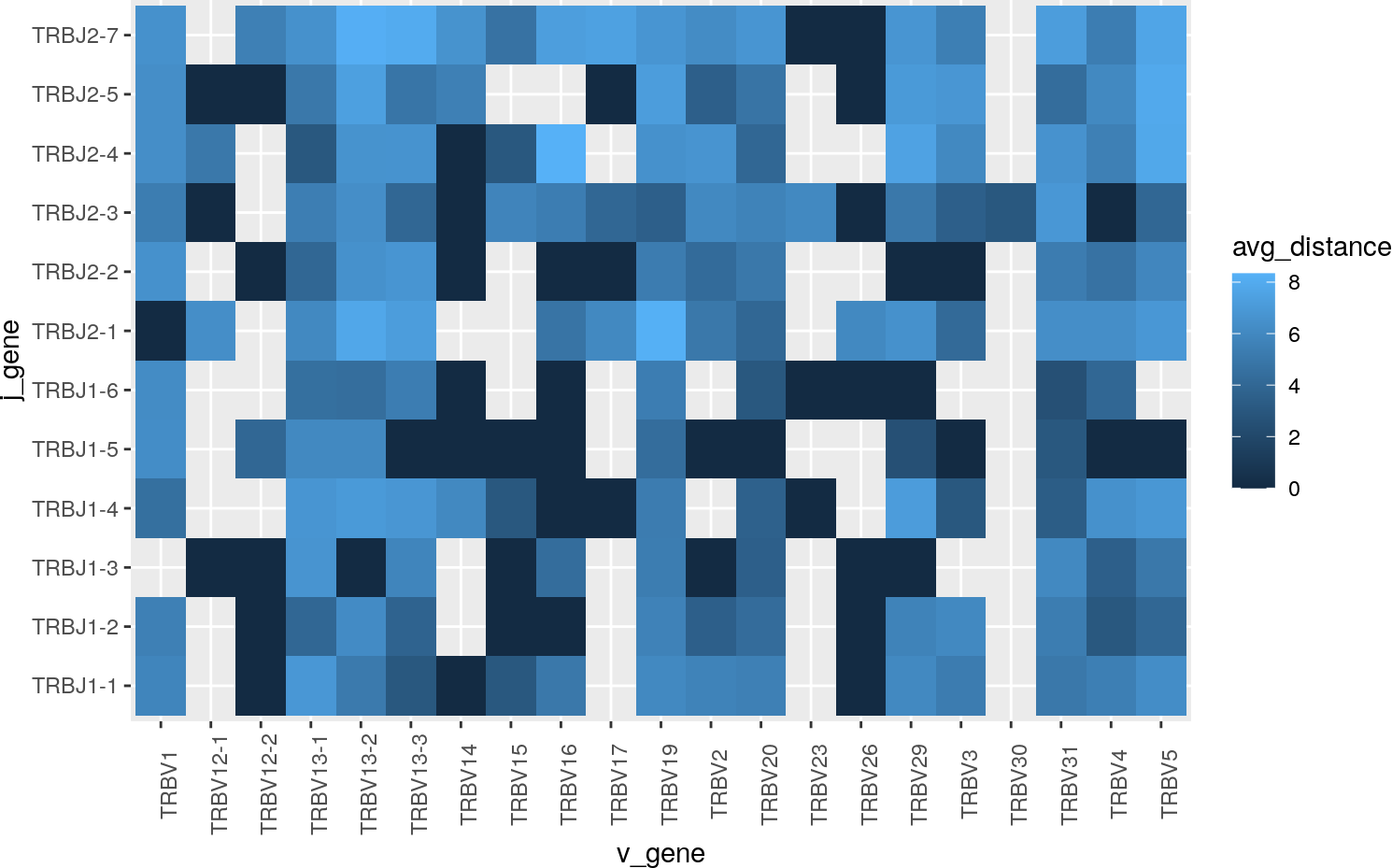Average Levenshtein distance of CDR3 within each pair. This might be turned into a z-score by fitting a weighted linear model with sum-to-zero contrasts and returning the pearson residuals. This could determine if a pairing has an unexpected small, or large, within cluster distance.

## 2.3 Expanded clusters

Next, we will examine the clusters that are found in many contigs. First we will get a canonical contig to represent each cluster. This will be the medoid contig, by default.

``````aa80 = canonicalize_cluster(aa80, representative = 'cdr3', contig_fields = c('cdr3', 'cdr3_nt', 'chain', 'v_gene', 'd_gene', 'j_gene'))
#> Filtering `contig_tbl` by `is_medoid`, override by setting `contig_filter_args == TRUE```````

`aa80` now includes the fields listed in `contig_fields` in the `cluster_tbl`, using the values found in the medoid contig.

``````MIN_OLIGO = 7
oligo_clusters = filter(aa80\$cluster_tbl, n_cluster >= MIN_OLIGO)
oligo_contigs = aa80
oligo_contigs\$contig_tbl = semi_join(oligo_contigs\$contig_tbl, oligo_clusters, by = 'aa80')
oligo_contigs
#> ContigCellDB of 54 contigs; 832 cells; and 4 clusters.
#> Contigs keyed by barcode, pop, sample, contig_id; cells keyed by barcode, pop, sample.``````

Get contigs/cells/clusters found at least 7 times (across contigs). Note that replacing `contig_tbl` with the subset selected with the `semi_join` also automatically subsetted the `cell_tbl` and `cluster_tbl`.

``````oligo_clusters = oligo_contigs\$contig_tbl %>% group_by(aa80) %>% summarize(`n subjects observed` = length(unique(sample))) %>% left_join(oligo_clusters)
#> `summarise()` ungrouping output (override with `.groups` argument)
#> Joining, by = "aa80"

knitr::kable(oligo_clusters %>% select(aa80:cdr3, chain:j_gene, avg_distance, n_cluster))``````
aa80 n subjects observed cdr3 chain v_gene d_gene j_gene avg_distance n_cluster
111 6 CVVGDRGSALGRLHF TRA TRAV11 None TRAJ18 0.6071429 28
172 5 CAVSRASSGSWQLIF TRA TRAV9N-3 None TRAJ22 2.1111111 9
296 6 CAASASSGSWQLIF TRA TRAV14D-2 None TRAJ22 1.5000000 8
808 4 CATGNYAQGLTF TRA TRAV8D-2 None TRAJ26 1.3333333 9

Report some statistics about these expanded clusters, such as how often they are found, how many subjects, etc.

``````oligo_plot = ggplot(oligo_contigs\$contig_tbl, aes(x = representative, fill = chain)) + geom_bar() + coord_flip() + scale_fill_brewer(type = 'qual') + theme_minimal()
oligo_plot``````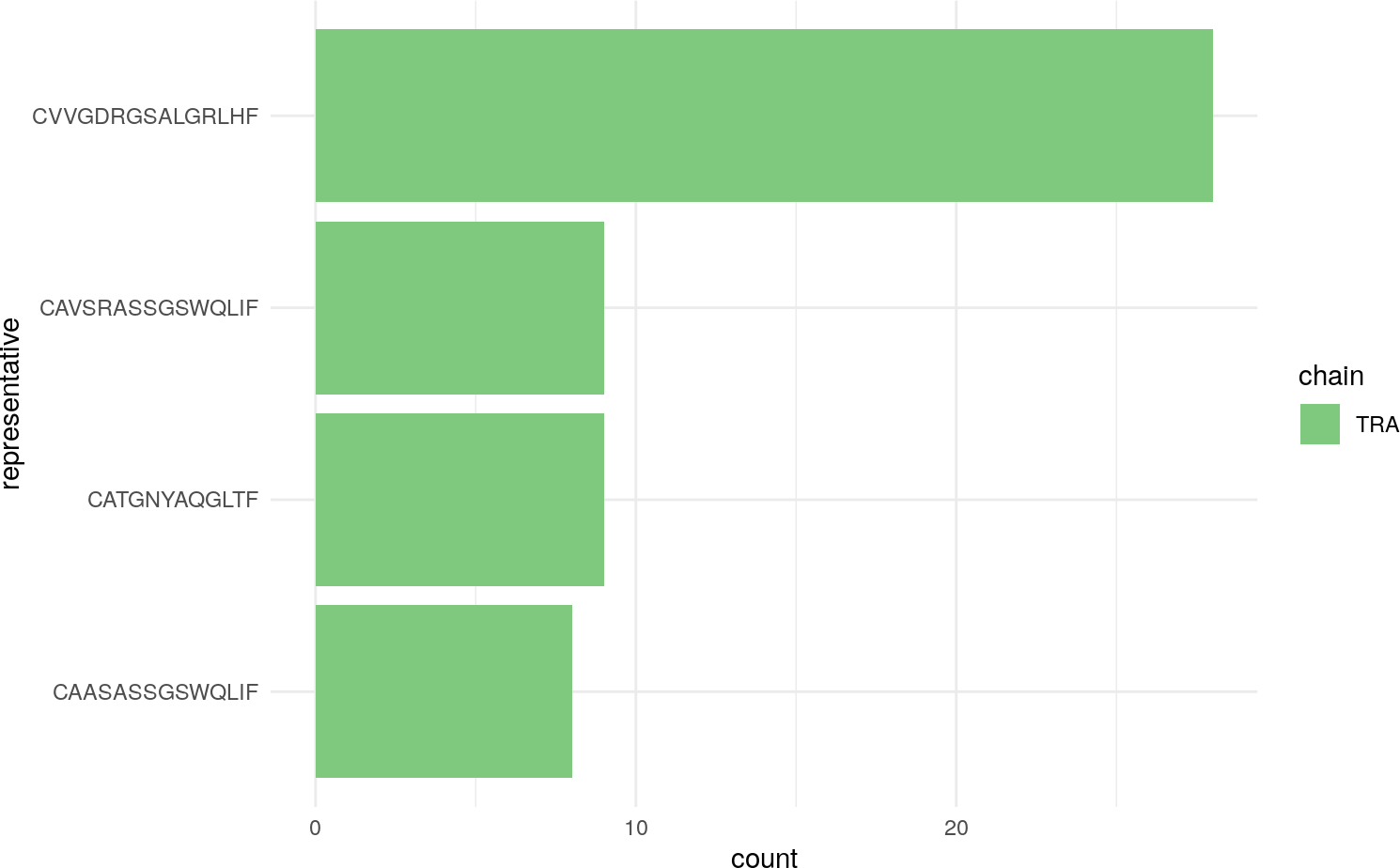These always come from a single chain.

``oligo_plot + aes(fill =   sample) + facet_wrap(~pop)``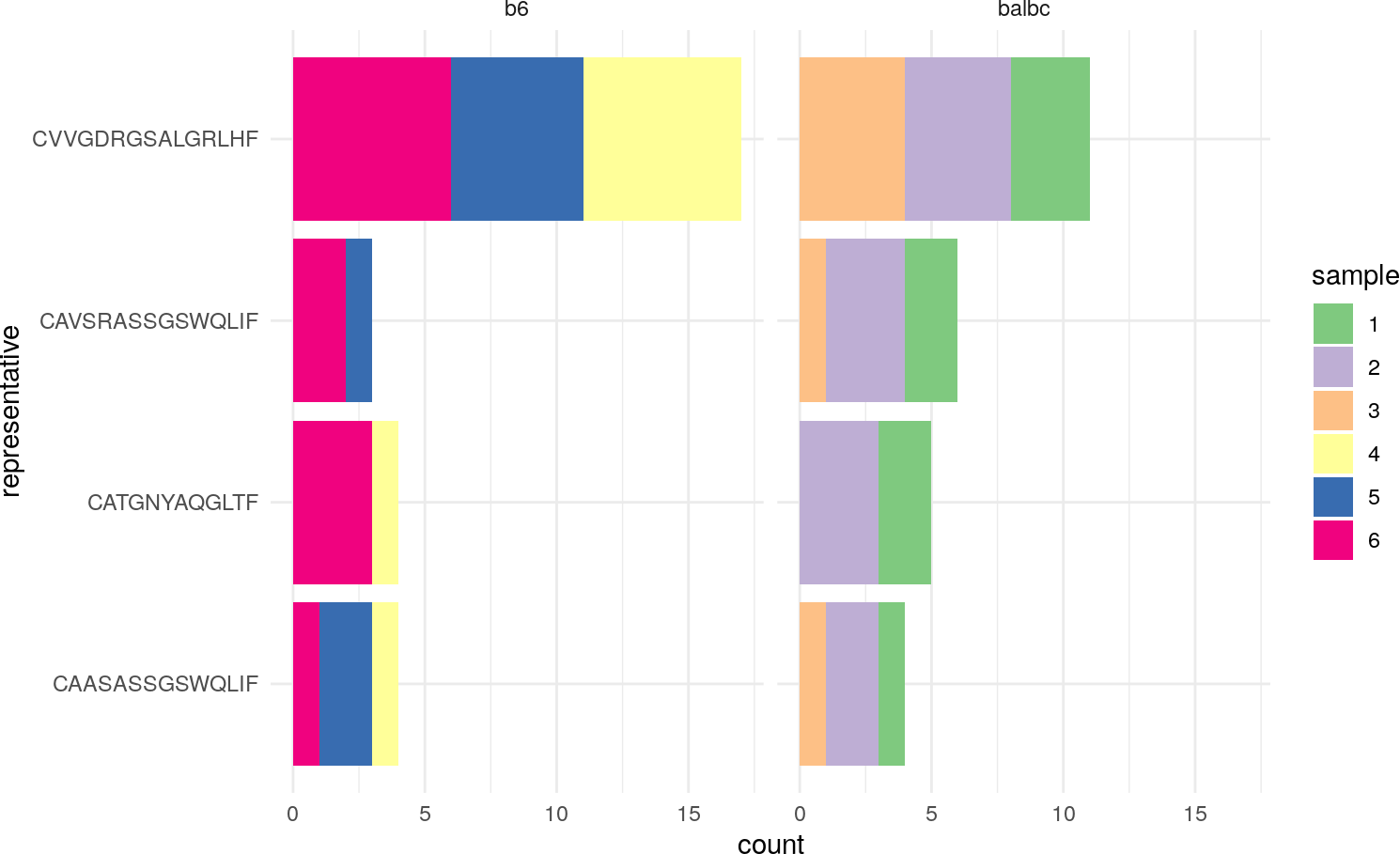But come from multiple populations and samples.

## 2.4 Some simple phylogenetic relationships

By using the within-cluster distances, some rudamentory plots attempting to show phylogenetic associations are possible. (These are most biologically appropriate for B cells that undergo somatic hypermutation.)

``````library(ggdendro)

dendro_plot = function(ccdb, idx, method = 'complete'){
h = filter(ccdb\$cluster_tbl, !!sym(ccdb\$cluster_pk) == idx) %>% pull(fc) %>% .[]
quer = filter(ccdb\$contig_tbl, !!sym(ccdb\$cluster_pk) == idx)
hc = hclust(as.dist(h\$distance_mat), method = method) %>% dendro_data(type = "rectangle")
hc\$labels = cbind(hc\$labels, quer)
ggplot(hc\$segments, aes(x=x, y=y)) + geom_segment(aes(xend=xend, yend=yend)) +
theme_classic() + geom_text(data = hc\$labels, aes(color = sample, label = fancy_name), size = 3, angle = 60, hjust =0, vjust = 0) + scale_x_continuous(breaks = NULL) + ylab('AA Distance') + xlab('')
}

to_plot = aa80\$cluster_tbl %>% filter(min_rank(-n_cluster) == 1)

map(to_plot\$aa80, ~ dendro_plot(aa80, .))
#> []``````